You’re completing your maths, chemistry or physics homework and are flying through all the questions. You’re super confident with the formulas and feel fully prepared for the test.

You walk into the classroom ready to go, you read a question and …. “what the flying spaghetti??” you have never seen anything like it before. You have no idea where to begin… help!

You are not alone! Maths, chemistry and physics present a specific challenge through worded questions that includes deciding which formula to apply.

In most cases you can use a simple five step process to break down worded questions and decide which formula applies: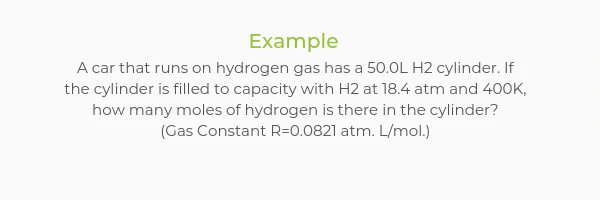Step One
Identify the known variables
The known variables are the values that are given to you in the question. Go through the questions and identify and label each given value with its corresponding variable.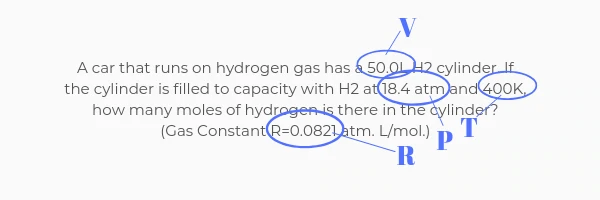Step Two
Identify the unknown variables
The unknown variable is what the question is asking you to find/solve. Identify the unknown and label it with its corresponding variable.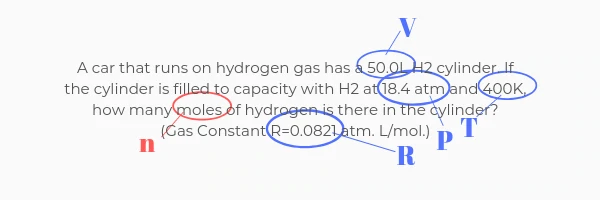Step Three
Decide which formula is relevant
Using the formulas you know, decide which one links all the known and unknown variables together.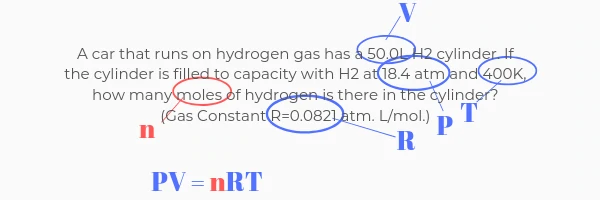Step Four
Rearrange the formula
Using algebraic principles, rearrange the formula to make the unknown variable the subject.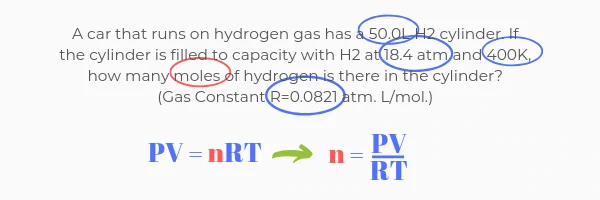Step Five
Solve for the unknown
Substitute the known values into the formula to solve the unknown variable. Make sure you check the units are correct in the question and in your answers!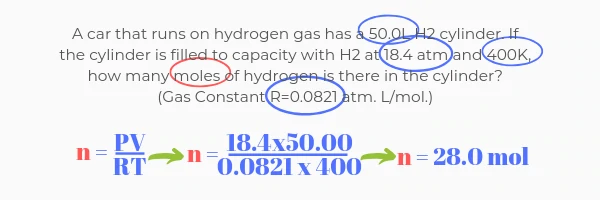For more help with identifying which formula to use in worded questions email us at advice@truecoaching.com.au